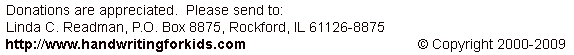Addition: Level 2: Exercise 4 (no carryovers)

Enter your answers in the boxes then click on SUBMIT:

 49+0 11+5 28+1 96+3 20+7 36+2 63+6 34+3 15+4 41+6 51+8 15+3 42+1 16+2 32+7 70+4 22+6 65+2 80+9 74+4 83+1 55+2 10+8 45+3 21+6 81+7 14+2 54+4 38+1 64+3